# How to Fix the Formula Error in Excel

## How to Solve Formula Error in Excel?

When Excel can not properly evaluate the formula in the spreadsheet, it will display Formula Error. The error message displayed gives us information about the type of formula error and what is the reason for the formula error. Formula Error breaks the flow of the whole spreadsheet. When the reports are displayed, the error may actually be disturbed.

This tutorial will help you to know what each formula error in the spreadsheet is. Why such error is there and how can you remove those formula errors in the Excel spreadsheet? When we enter some Formula in the spreadsheet, we will learn to handle some error that can be seen in Excel spreadsheets.

### Types of Formula Error are as follow:

1. #NULL! – Formula Error in Excel

This error means the wrongly Cell References This error is the least seen in Excel spreadsheets.

Reason of #NULL! Error in Excel –

>> Cells are referring to two areas of Excel Spreadsheet that do not distinguish one another.

>> When entering the formula, two or more cells are unknowingly separated.

Avoid this Error –

>> Separate cell reference by mathematical operator.

>> The beginning and end of the cell reference should be separated with the colon mark (:)

>> Personal cell references separate from a comma.

Example of #NULL! Error in Excel –

In the example we have taken cell range A1:A5 and C1:C5. When searching the minimum value in cell E2, we found error #NULL! This error occurs as the error occurs because two categories of cells do not distinguish each other in the Excel spreadsheet.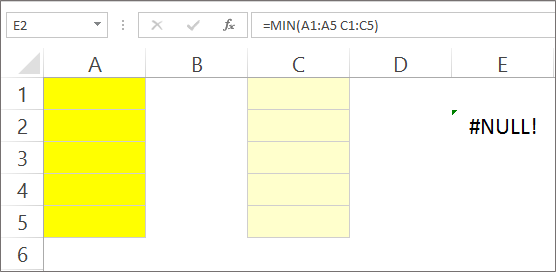Looking at the same example, if we have changed cell references then the result will return a price. Here we changed our cell reference from A1:A5, C1:C5 to A1:A5, A1:C1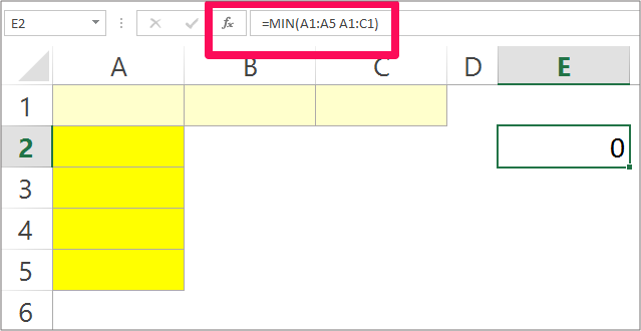We get results after changing cell reference is 0. In short, we are #NULL! Instead of getting an error that appears in the first image of the function, return a value for the function.

2. #REF! – Formula error in excel

This error is called a reference error. This means formula is referring to an invalid cell.

Reason of #REF! error in excel –

>> Any of the lines or columns mentioned in the formula has either been removed or the data has been transferred to another cell which has not been referred.

>> A link in the cell that is no longer working.

>> The references mentioned in the formula can not be updated automatically.

Avoid this Error –

>> Use the Excel Undo feature or press the Excel shortcut key Ctrl + Z to recover lost data.

>> Adjust cell references or re-enter data in cell

>> In place of the second link, update the cell with a working link which has stopped working.

Example of #REF! error in excel –

Here we will calculate Sum Formula for a series of cells. Sum formula is displayed in cell C6 for B2:B4, C2:C4, D2:D4.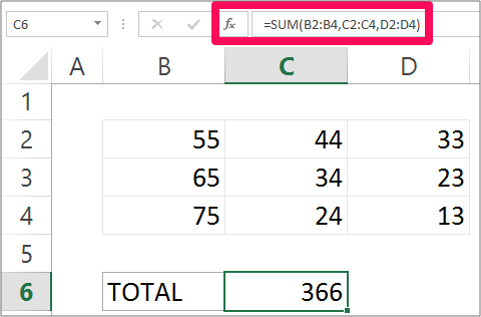Take the same example and keep the same formula, we remove the column D from the example. In the Formulas tab we see that instead of cell reference D2:D4, let us #REF! Get.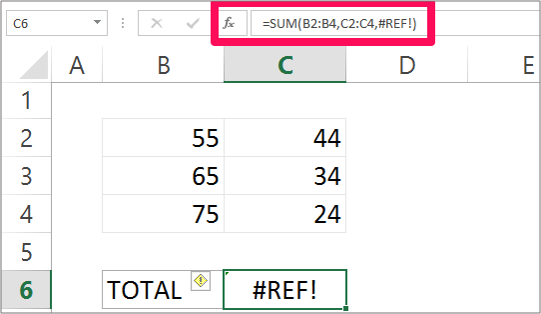The result is a formula error #REF! That’s because we have removed the data from column D We have not changed our logic limit in the formula. If we have changed the arguments, then we’re #REF! Instead of returning a price.

3. #DIV/0! – Formula error in excel

The error itself says that anything divided by zero or empty cells will return an error. It’s easy to solve this error.

Reason of #DIV/0! Error in excel –

>> Divide a number by zero or an empty cell.

>> Either the cell is empty or we try to divide it by zero.

>> In other words, the separator and denominator are both zero or empty cells.

Avoid this error –

>> Check that the data is entered correctly in the cell.

>> Check whether the correct reference is given in the formula or not.

>> Restore your data by using the undo option or pressing the shortcut CTRL + Z.

Example of #DIV/0! error in excel –

Here we take two examples. In the first example we will take numbers and in the second example we will refer to the cell to find the error. In the first example, we try to divide 45 to 0 in the cell and we call the value to #div/0! Let’s back as! Error.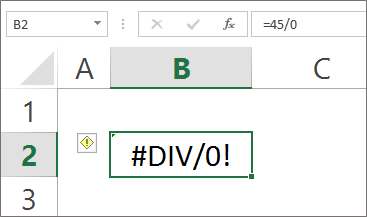In the second example, we are referencing cell reference. In cell D3 we enter = B3/C3 and press Enter.For example we get results # DIV/0! Error. This is because in the first example we are dividing it by zero and in the second example we are dividing by an empty cell.

4. #### – Formula error in excel

You see a cell full of Hashtags Character. This is not really an error.

Reason of #### error in excel –

>> The column inside the cell is not wild enough to display.

>> The date and time in the cell are in negative value.

Avoid this error –

>> Width the column by either double-right to the right of the column or drag the column headers.

>> Or reduce the value in the cell by reducing the decimal button in the Home tab> Number group.

Example of #### Error in excel –

We have taken a long number of 927465892947463819 to enter the small cell, what do we see?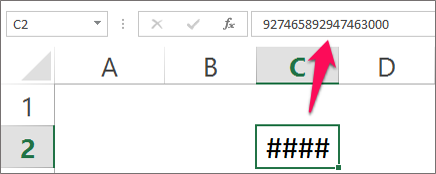The result value is ####. This is because cell size is not so large to show the number that has been entered.

5. #NAME? – Formula error in excel

The error does not recognize the text in the formula. This error does not recognize the name of the formula that we are trying to work on. This error is a bit complicated.

Reason of #NAME? error in excel –

>> The name of the excel function was not recognized.

>> Do not read some elements of Excel formula.

>> Formula arguments can be entered incorrectly.

Avoid this error –

>> Type the correct spelling of the formula.

>> Give correct cell reference of the boundary.

>> Range should be defined only by using the colon.

Example of #NAME? error in excel –

Here we want to get maximum value from different values ​​given in different cells. Therefore we enter the function as =MAX(B2: F2). Well what do we see that we call the value in cell #NAME? Return as an error.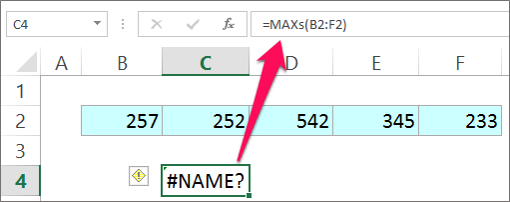Did you see what happened now? You might be wondering what went wrong? Well we missed the MAX function incorrectly. The correct spelling is MAX instead of MAXS. Correct the spelling and press enter.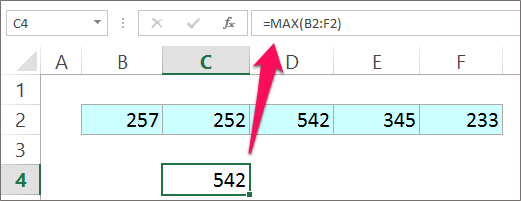The result is a value to get us. But it was possible only after correcting our spelling error. Otherwise our value is #NAME? Error will be there.

6. #N/A – Formula error in excel

This error means that the numbers can not be found in Formula.

Reason of #N/A error in excel –

>> No price has been found.

>> Inappropriate logic has been entered in the formula.

>> A sheet that we are referring to has either been removed or not saved.

Avoid this error –

>> The arguments recorded in the formula are correctly referenced.

>> Check that the entered arguments have not been deleted.

>> Do not use N/A or NA as part of the data.

>> See that any data in the logic is missing.

Example of #N/A error in excel –

In the example below, we have a list of the account holder name along with the bank holder, check number, account number and amount.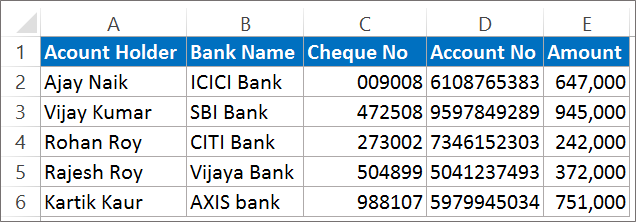We want to find the check number and the amount of that check for some of the names below. We use VLOOKUP formula to search the check number and amount of the specific account holder.

But, whatever we return is an error. A #N/A error that is not vertical for the value.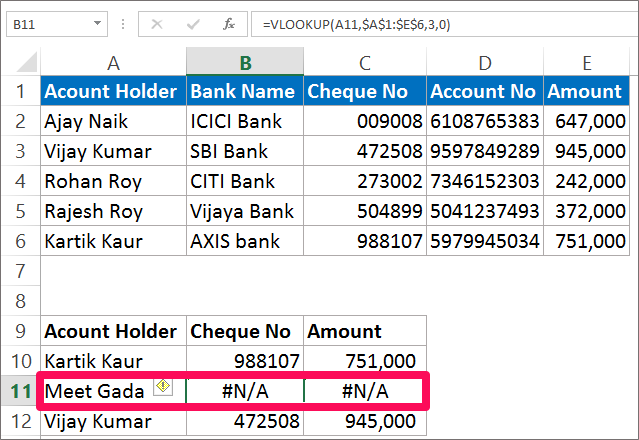We get the result as error because the account holder does not have the name Meet Gada.

7. #NUM! – Formula error in excel

This error means the numerical values ​​that are not valid. In other words, the invalid numbers are in the formula.

Reason of #NUM! error in excel –

>> The result number is too large to handle Excel.

>> Entering numerical values ​​different from another value.

>> Entering the wrong data type in the function.

>> Using negative values ​​to find square root will result in this error.

Avoid this error –

>> Enter a correct type of function.

>> Avoid using negative values.

Example of #NUM! error in excel –

Here we will find square root for the number-15 using square root formula. The number recorded in cell B2 is negative.

Now, enter the SQRT formula in cell B3. =SQRT(B1) Press enter.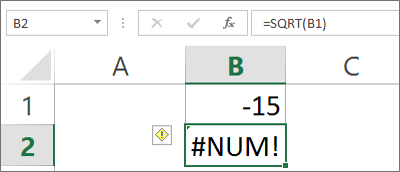Answer us #NUM! This is because error is returned because the square root of the negative number will return this error. Now changing the value from negative to positive will give us a value.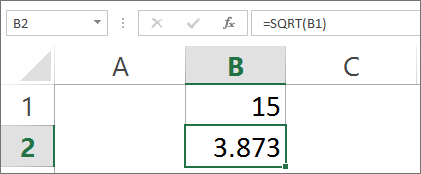8. #VALUE! – formula error in excel

This error indicates that something entered or typed in the formula is incorrect. This is a common error and it is most difficult to find the exact reason behind error.

Reason of #VALUE! error in excel –

>> Wrong logic has been entered in the formula.

>> Wrong place, text, character entered instead of number.

>> When it is given and does not match what is expected.

Avoid this error –

>> Double-check the formula that you have entered the correct number.

>> Check that no empty cell is entered in the argument or not.

>> Also, see that no particular character has been entered in arguments.

Example of #VALUE! error in excel –

In the example we will add value to 3 cells i.e. cell B2, cell C2, and cell D2. We will add these 3 cells individually to cell C4. For example, we will enter cell =B2+C2+D2 and press enter.

Note: If we use Sum Function, it will return a value.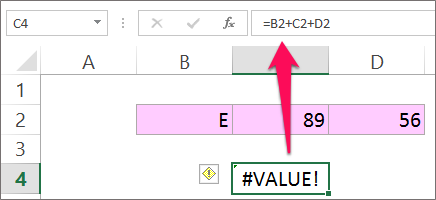Keeping the same example in mind, changing the cell value from B to a number from E Let’s take 37.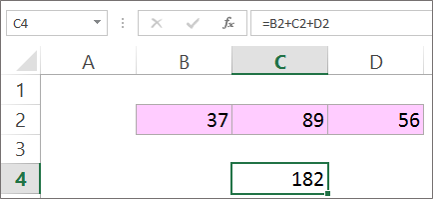What will we see that we will get a price in return. The answer we get is 182. Here the error prevails if we change the cell value of B2 or we use Sum Formula.

9. #GETTING_DATA – Formula error in excel

This error is also not a real error. This is a message that appears in Excel spreadsheets. This is like a status report.

Reason of #GETTING_DATA error in excel –

>> A message is popping up due to the large complex data count.

>> Different operations are grouped in the cell and complex data is entered.

>> While the calculation is still in progress, incomplete cells will display an error.

Avoid this error –

>> You can not escape from this error.

>> As soon as unfinished work, this error will disappear. This is the reason why this error is called a temporary error.

Hope you like our tutorial for more cool and amazing trick of excel Like our Excel Superstar Facebook Page and subscribe to our Excel Superstar YouTube channel. Excel Superstar is the leading online training company, which provides Online Excel Course in Hindi. Connect with us and become an Excel Superstar.